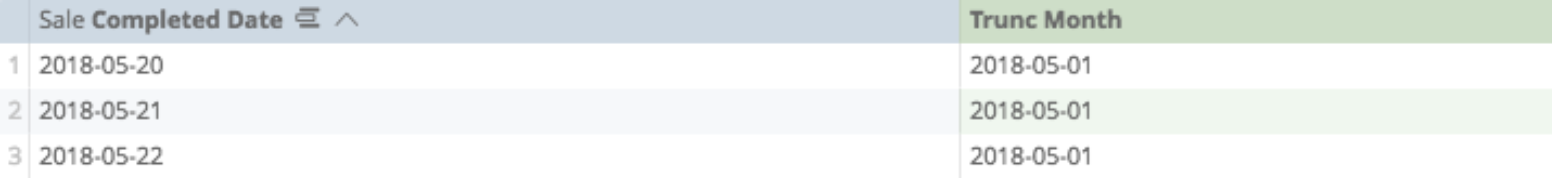# Date functions

Analytics provides many functions that perform different mathematical, logical, and table-based calculations. You can find a more in-depth catalog of available functions on the Looker functions and operators page.

To make it easier, these are categorized in five different ways:

## Add_Days (or Weeks, Months, Years)

This looks at a date field and then changes it by a defined number (one day is equivalent to the number 1).

• The first argument is the number difference, which can be positive or negative.
• The second argument is the date that you wish to adjust.

In this example, there are two calculations — one to change the sales day forward by one day, the second to change the sales day backward by one day: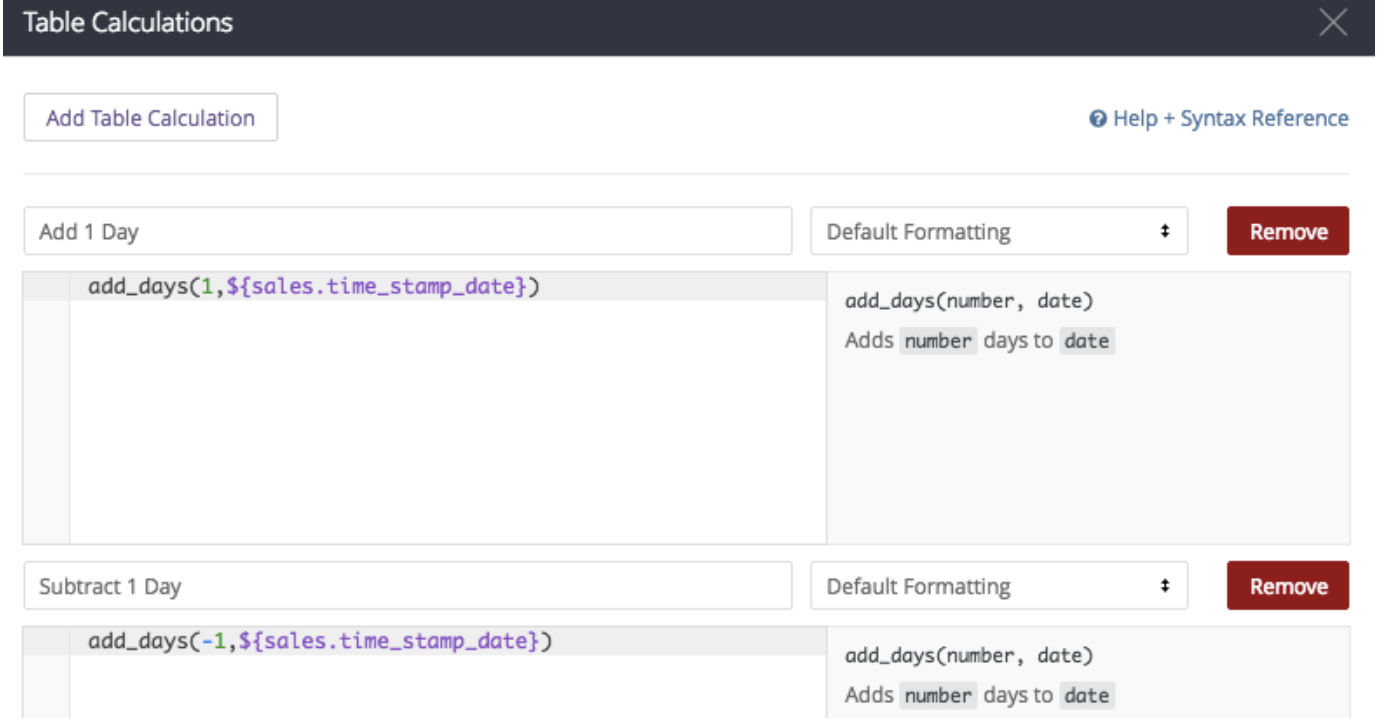The calculations are as follows:

If built properly, here are the results: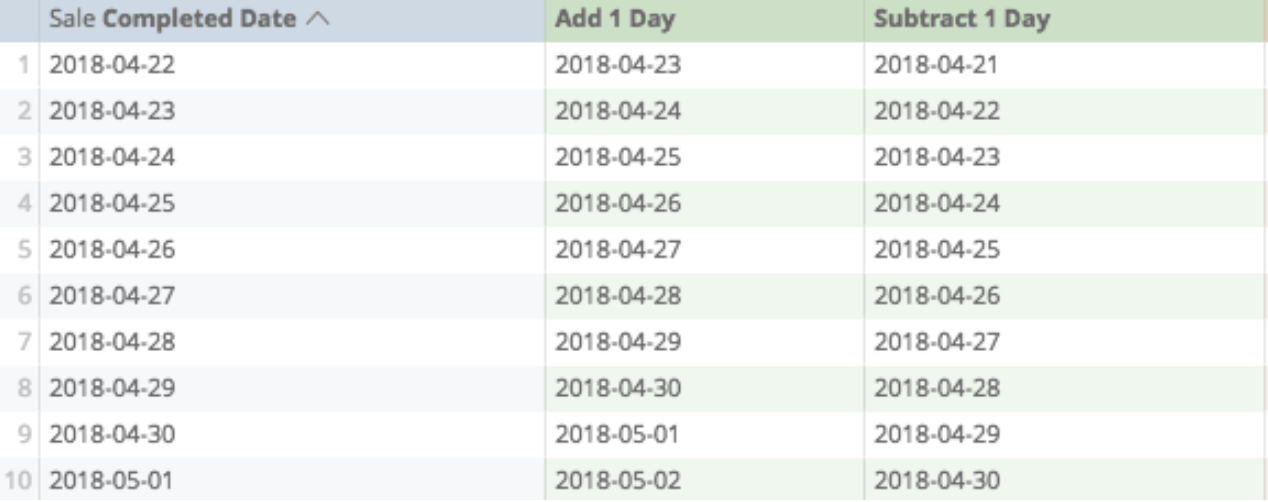## Date

This creates a date out of provided numbers, which requires three arguments:

• The first argument is the number for the year.
• The second argument is the number of the month (the number one corresponding to January, the number two corresponding to February, and so on).
• The third argument is the number of the day.

Example date calculation: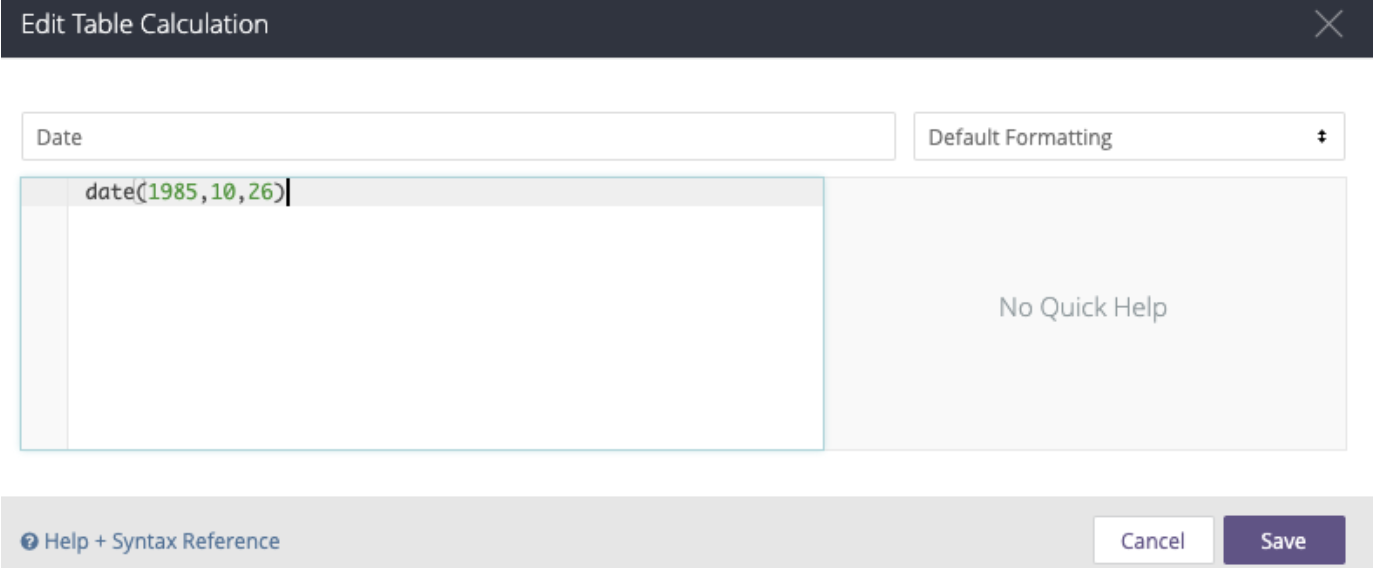Example result: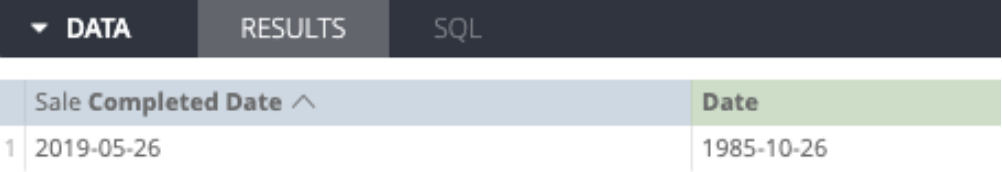## Diff_Days (or Weeks, Months, Years)

This counts the difference in days (or other denominations) between two dates.

To prepare it, the first argument is the start date (or earlier date), and the second is the end date (or later date).

Note: This logic is different from how spreadsheets may calculate differences between dates, where you might subtract a smaller from a larger to yield a positive number.

If you want to count the difference from the start of the month to the current sales date, prepare a calculation like this: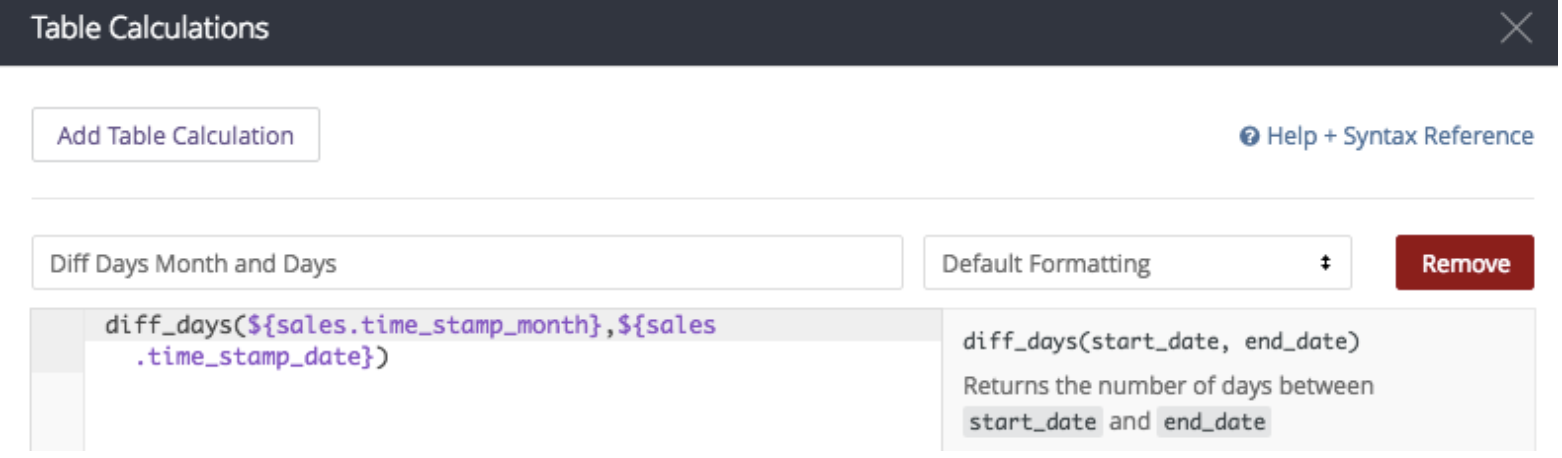The calculation:

• diff_days(\${sales.time_stamp_month},\${sales.time_stamp_date})

And if done properly, here is the result: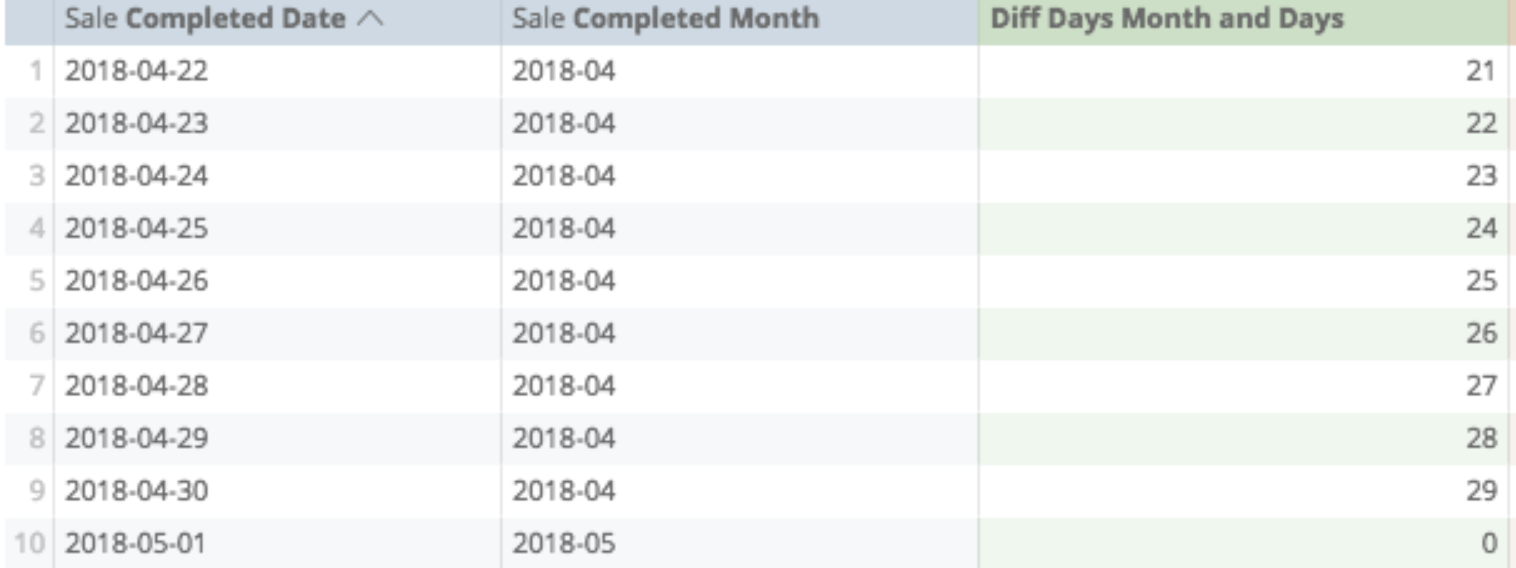## Extract_days (or Months, Years)

This converts part of a date to a non-date number.

#### Example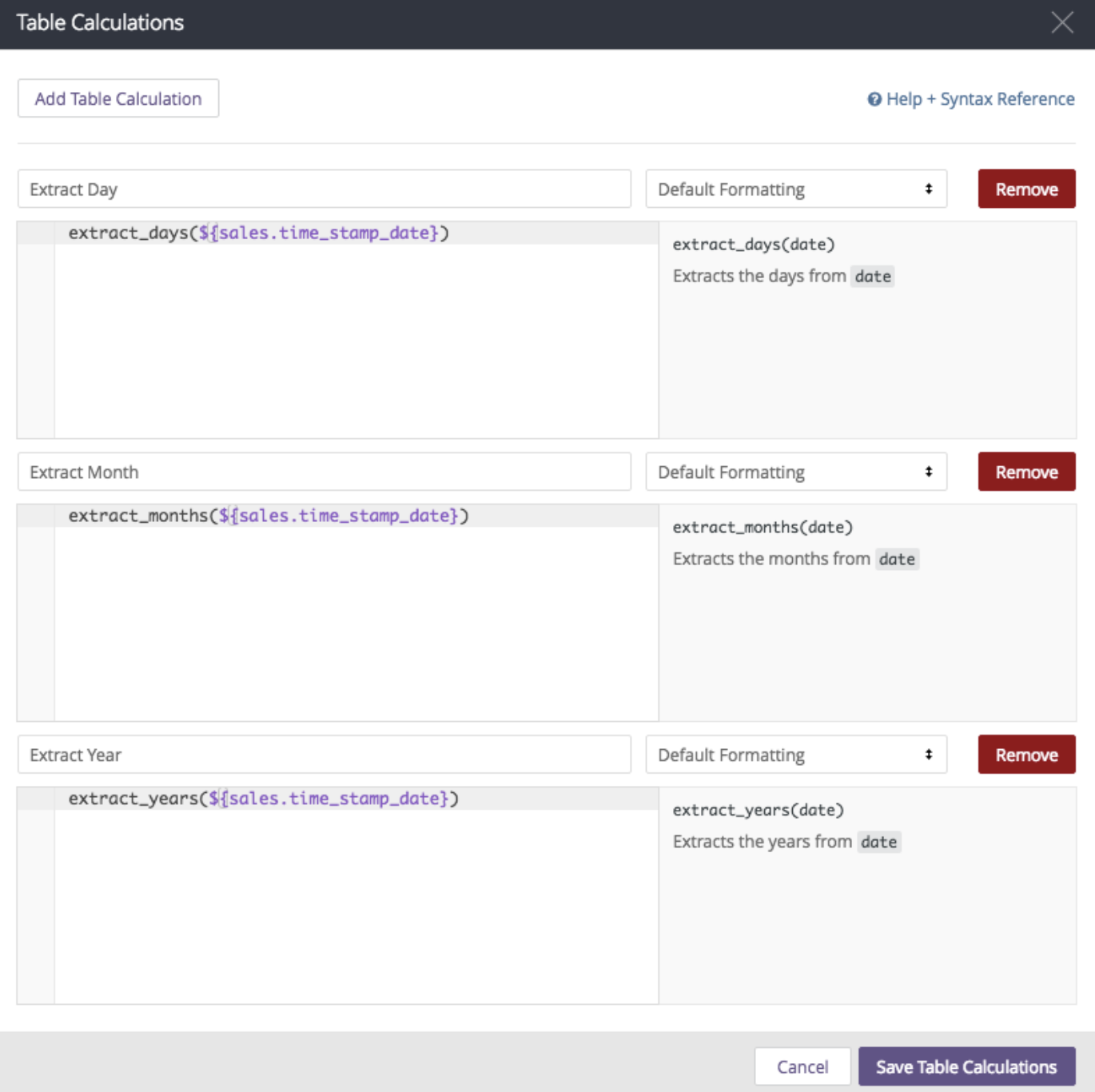The calculations:

• extract_days(\${sales.time_stamp_date})
• extract_months(\${sales.time_stamp_date})
• extract_years(\${sales.time_stamp_date})

If prepared properly, here is the result: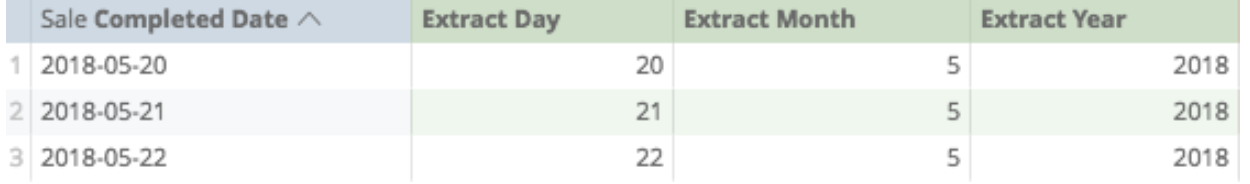## Now

This returns the current timestamp; no other arguments are required.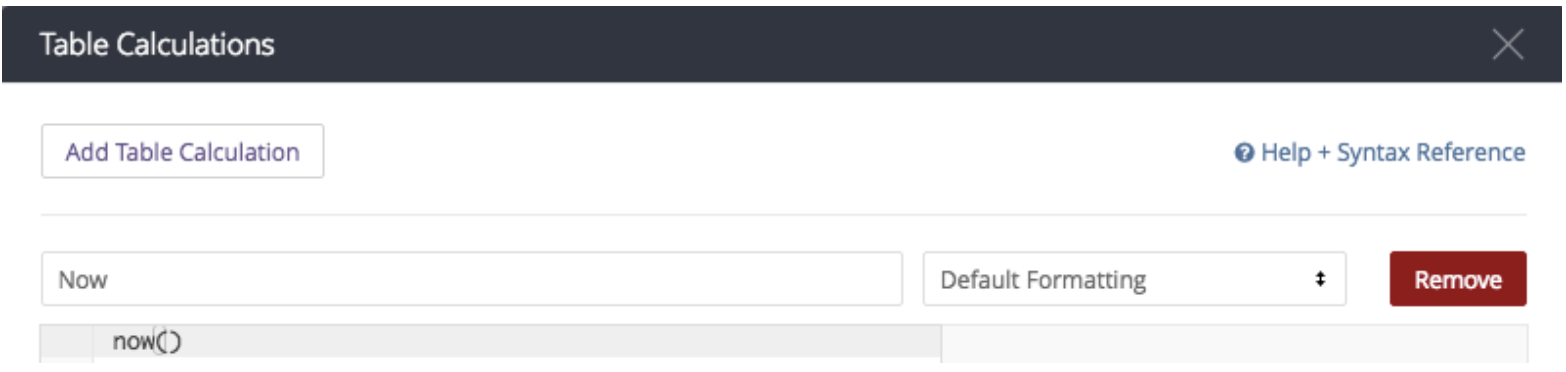In this instance, here's the calculation: now()

If saved properly, here is the result: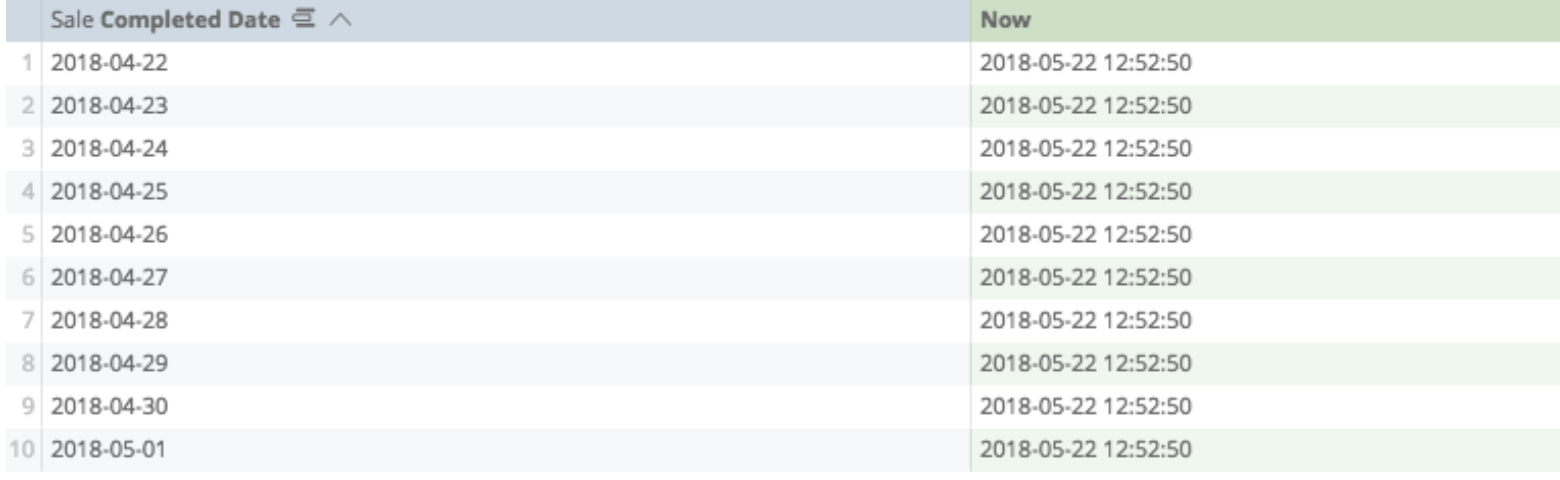## To_date

Changes a string of text numbers to a date, and must follow one of the following formats:

• YYYY
• YYYY-MM
• YYYY-MM-DD
• YYYY-MM-DD hh
• YYYY-MM-DD hh:mm
• YYYY-MM-DD hh:mm:ss
Note: The year 2018, the number 2,018, and the word 2018 are not considered the same by Analytics. In this instance, it is the word 2018 that responds to the to_date function.

In this calculation, the date was created from straight text: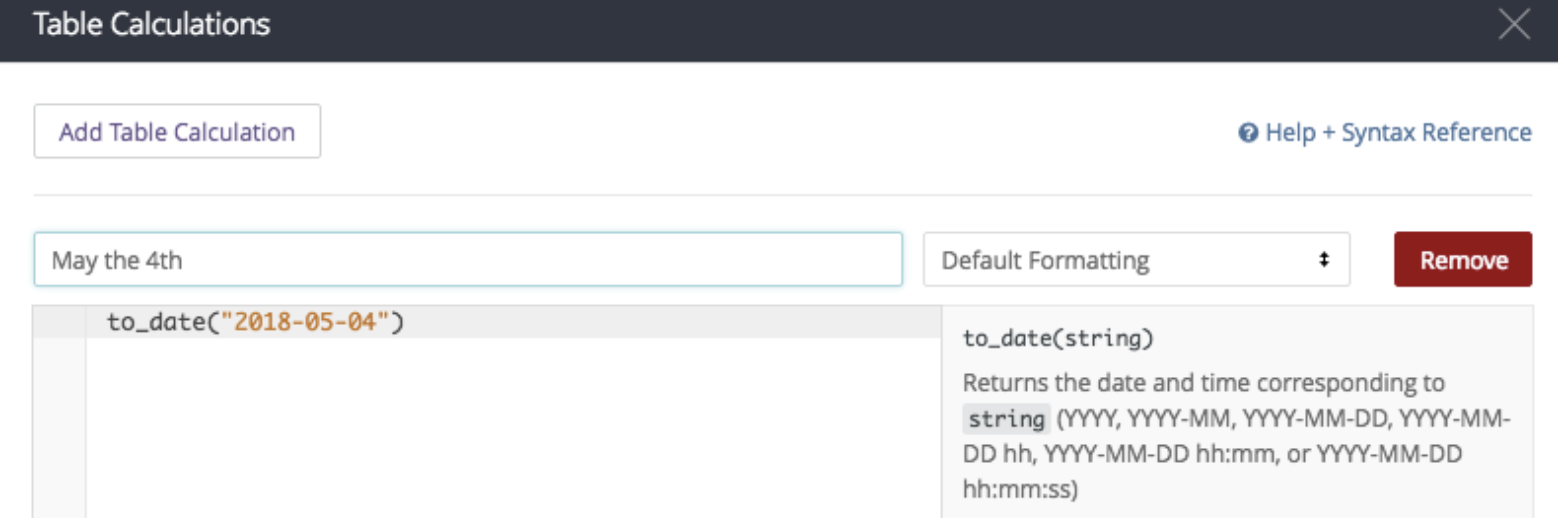The calculation: to_date("2018-05-04")

The results: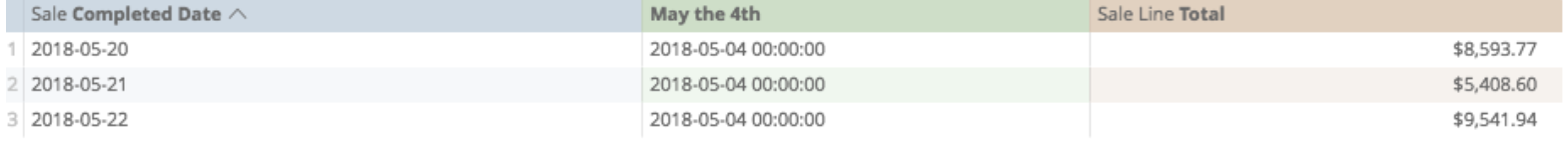## Trunc_Days (or Weeks, Months, Years)

This rounds time down to the desired closest interval. In this instance, the trunc_months function changes the sale date to the month: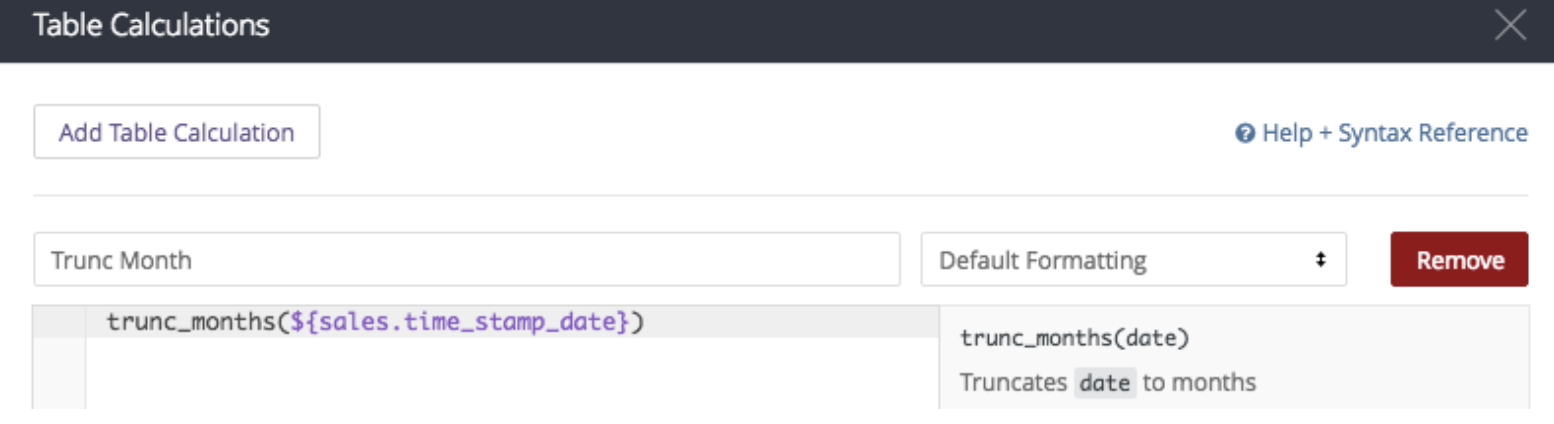The calculation: trunc_months(\${sales.time_stamp_date})

If prepared properly, here are the results: